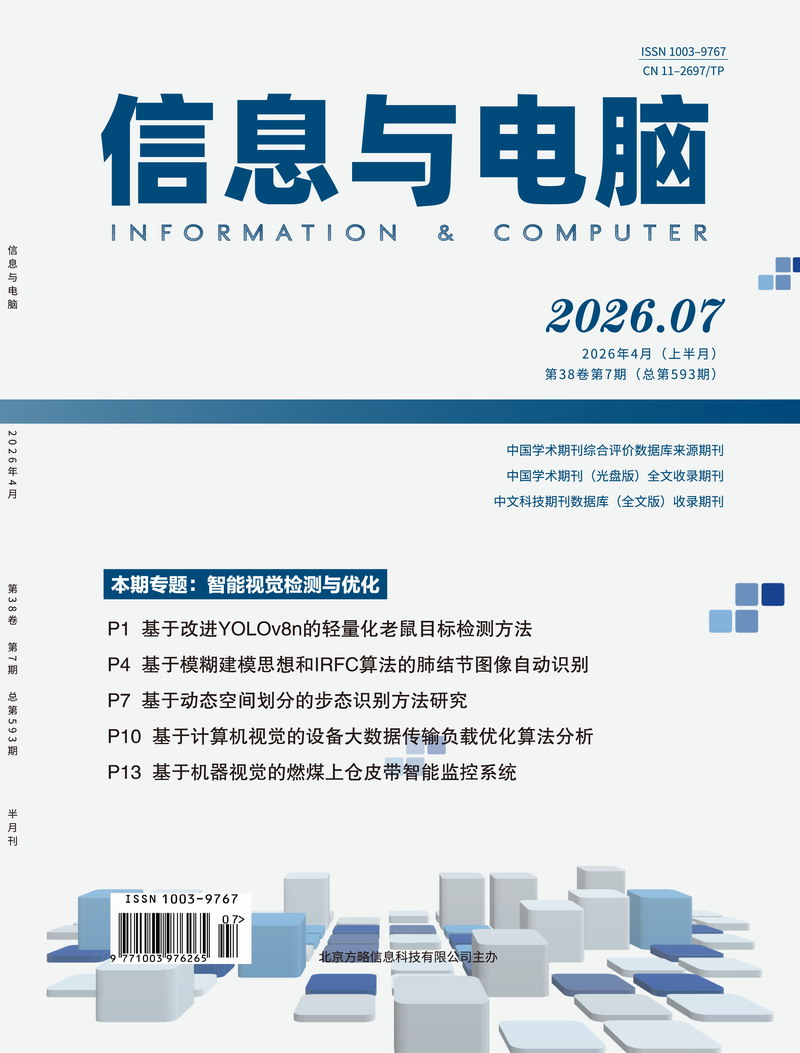# 《信息与电脑》录用通知

## 您的当前位置：首页 >> 录用通知

单模型机动目标跟踪算法仿真

:  本文针对单模型的机动目标，具体阐述了匀加速模型（CA）的应用实例，通过算法仿真分析出这两个模型在实际应用中的优缺点，并且指出CV模型应用的局限性，以及CA模型在加速度跳变的时刻存在一个收敛的过程，当达到收敛时其在加速阶段的跟踪效果比在匀速阶段跟踪的效果要好这一突出特点，为以后通过改善跟踪门来解决单模型机动目标跟踪性能的方案提供参考依据。

1    提出问题及场景假设

1.1 提出描述

1.2、场景假设

2    理论模型及模型算法

CV模型可以表示为：

CA模型可以表示为：

3    具体实现

CA(匀加速)模型

取状态变量：

X(k+1)=ΦX(k)

Z(k)=HX(k)+V(k)

Z=             V=

H=              Φ=

(k/k-1)=Φ(k-1/k-1)

P(k/k-1)=ΦP(k-1/k-1)ΦT

K(k)= P(k/k-1)HT[H P(k/k-1)HT+R]-1

(k/k)=(k/k-1)+K(k)[Z(k)-H(k/k-1)]

P(k/k)=[I-K(k)H]P(k/k-1)

R=

在应用实践中，我们通常无法知道目标的初始状态，那么我们可以使用前几个观察来建立状态的初始估计。由于仅考虑了目标位置和速度，因此这里使用前两个观测值来建立初始估计，即两点起动方法：

(2/2)=

==10000

(k-Δ/k-Δ)=     (k-Δ/k-Δ)=2/T     (k-Δ/k-Δ)=2/T2

(k-Δ/k-Δ)=4[+(k-Δ-1/k-Δ-1)+2T(k-Δ-1/k-Δ-1)+T2(k-Δ-1/k-Δ-1)]/ T4

(k-Δ/k-Δ)= 4/T4+4(k-Δ-1/k-Δ-1)/T2+(k-Δ-1/k-Δ-1)+4(k-Δ-1/k-Δ-1)/T

(k-Δ/k-Δ)= 4/T3+4(k-Δ-1/k-Δ-1)/T3+2(k-Δ-1/k-Δ-1)/T+6(k-Δ-1/k-Δ-1)/T2

4    仿真结果

CA 模型（左）和singer模型（右）

1 目标速度变化情况

2 目标加速度变化情况

2显示了CA模型的速度估计与速度的标准差，由图可知该算法在滤波开始时需要一定的收敛过程存在比较大的误差，并且在加速度突变时，也是存在一个收敛的过程，这与实际是相符合的。

5    结束语

6    参考文献

 王丽娜. 基于卡尔曼滤波的单模型目标跟踪算法的仿真研究[J]. 中国新通信.2016.

 刘楠. 基于机动目标跟踪模型的自适应滤波算法[D]. 浙江理工大学. 2016.

 张喜涛; 张安清; 梁栋; 牛治永. 改进的“当前”统计模型变采样率目标跟踪算法[J]. 电讯技术. 2014.

 刘瑞腾. 目标跟踪滤波方法研究[D]. 西安电子科技大学. 2018.

 高恩克.目标跟踪的算法研究[D]. 西安电子科技大学 2007.

 蒋敏. 基于分布式卡尔曼滤波的目标跟踪方法研究[D]. 哈尔滨工程大学. 2016.

 沈莹.机动目标跟踪算法与应用研究[D]. 西北工业大学 2007.

 孙微. 机动目标跟踪滤波算法研究[D]. 哈尔滨工程大学. 2017.